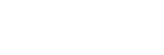## PHYSICS PAPER 3 - KCSE 2019 NYANDARUA PRE MOCK EXAMINATION

Question 1

1. You are provided with the following apparatus
• A metre rule
• A mass marked M
• Six 20g masses
• A stop watch
• A complete stand
• A balance for sharing

Arrange the apparatus as shown in the figure 1.1(a) below1. Attach the mass marked M to the free end of the spring to exert a downward force Mg in newtons as shown in figure 1.1(b) above. if the mass causes an extension L called static extension, then Mg=kL where k is the spring constant and g is acceleration due to gravity.
1. State the static extension L caused by M in cm
2. Using the balance, weigh the mass of M in grams …g                       (½mk)
3. Determine the spring constant k in Newton per meter if g=10.0ms-2
2. Pull the mass M down a further distance x below the equilibrium position as shown in figure 1.1c.   (x must be very small ≈5mm). Release the mass so as to oscillate up and down. Measure the time for 10 oscillations and record your values in the table shown below.
Repeat the experiment for the other values as shown and complete the table.             (6marks)
 Mass m(g) Extension L(cm) Time for 10 oscillations (s) Period T (s) T2 (S2) M M+20 M+40 M+60 M+80 M+100 M+120
3. Plot the graph of L (in meters) against T2                                  (5marks)
1. The slope of the graph
2. The intercept of the L – axis
4. If Ms is the effective mass of the spring, the period T of the oscillating system is given byUsing this formula calculate:
1. Acceleration due to gravity g
2. The effective mass Ms of the spring                                                                                                 (2mks)
1. You are provided with the following apparatus
• A voltmeter
• An ammeter
• A 1.5V dry cell
• Six pieces of connecting wires
• A meter rule
• Mounted resistance wire
1. Connect the voltmeter across the two dry cells on an open circuit as shown in figure 2.1 below.What is the reading of the voltmeter ………(1mk)
2. Now connect the dry cells to an external circuit in the form of a resistance wire by placing the jockey on the wire AB starting from L=20cm.Record the terminal p.d V in volts and the corresponding current I in amperes. Repeat the experiment for other values of L shown and complete the table.          (8mks)
 Length L (cm) Terminal p.d V(volts) Current I(A) R=V/I I/I (A-1) 20 30 40 50 60 70
3. Plot a graph of R against ⅟I       (5mks)
1. The slope      (2mks)
2. The intercept of the R axis           (1mk)
5. Given that R=(E. 1/1) -r
Where E is the emf of the cell, r is the internal resistance of the cell, Determine from the graph:
1. The emf E of the cell    (1mks)
2. The internal resistance r of the cell       (2mks)

#### Download PHYSICS PAPER 3 - KCSE 2019 NYANDARUA PRE MOCK EXAMINATION.

• ✔ To read offline at any time.
• ✔ To Print at your convenience
• ✔ Share Easily with Friends / Students